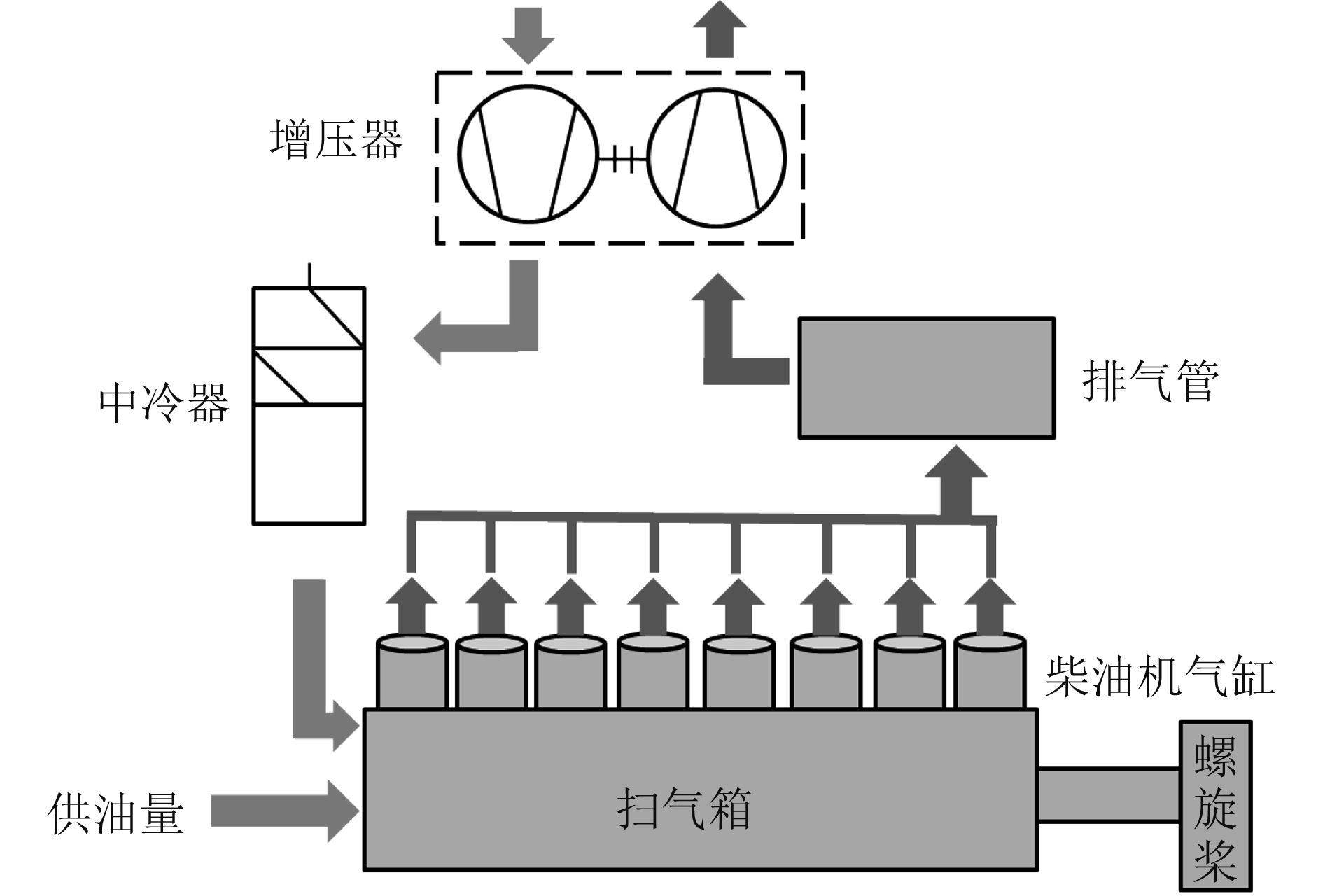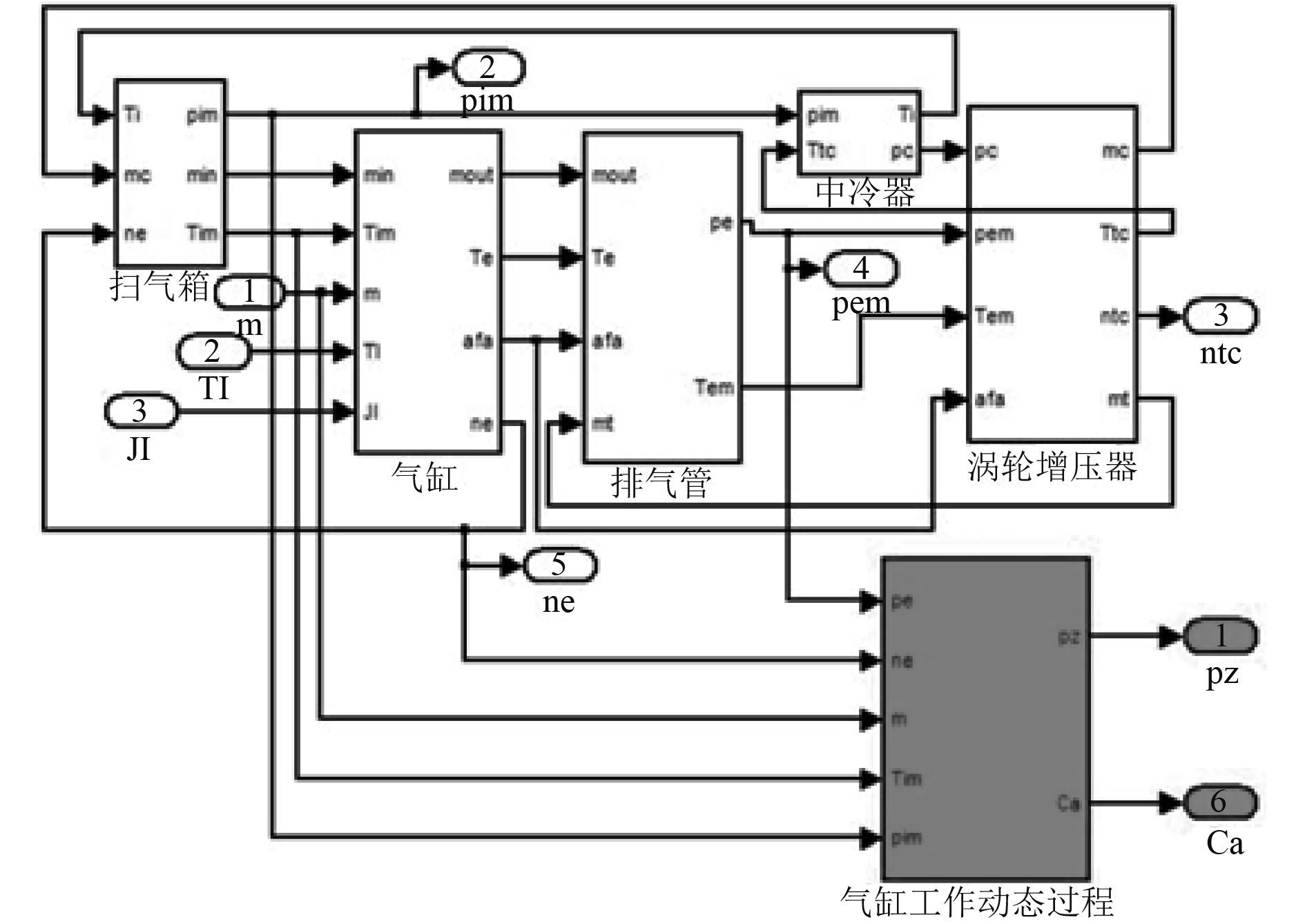﻿ 远洋船舶主机低负荷下燃烧工况优化研究
 舰船科学技术2018, Vol. 40Issue (3): 108-111PDF

The optimization research for main engine combustion conditions of the ocean vessel on low load
DONG Qing-feng, ZHANG Zhi-gang, LU Jia, GE Quan
China Satellite Maritime Tracking and Control Department, Jiangyin 214431, China
Abstract: This paper is conducting an optimization study on the special ships' serious issue during their main engine's combustion conditions under low load, firstly, I analyzed its combustion conditions and came up with the optimization solution of the constant speed mode, and I utilized the math model to certify the assumptions; then I conducted the engineering reform by applying the constant speed mode devices to those ships; in the end, I made a comparison of the performance parameters between the main engine's new mode and its original mode, I testify that the constant speed mode can effectively improve the combustion performance of the main engine.
Key words: low load     math model     constant speed mode
0 引　言

1 柴油机燃烧性能恶化分析

1）影响喷油器的性能

2）引起主机振动不均

3）影响船舶正常航行计划

2 柴油机数学模型的建立

2.1 平均值模型图 1 柴油机工作原理图 Fig. 1 Working principle of diesel engine

2.2 容积法模型

2.3 气缸工作过程基本方程
 $\begin{split}\frac{{{{d}}{T_z}}}{{{{d}}\varphi }} = \left( {\frac{{{{d}}{Q_f}}}{{{{d}}\varphi }} + \frac{{{{d}}{m_s}}}{{{{d}}\varphi }}{h_s} - \frac{{{{d}}{m_e}}}{{{{d}}\varphi }}{h_e} - } \right.\\\left. {\frac{{{{d}}{Q_w}}}{{{{d}}\varphi }} - {p_z}\frac{{{{d}}{V_z}}}{{{{d}}\varphi }} - {c_{vmz}}{T_z}\frac{{{{d}}{m_z}}}{{{{d}}\varphi }}} \right)\frac{1}{{{m_z}{c_{vz}}}}\text{，}\end{split}$ (1)
 ${p_z}{V_z} = {m_z}{R_z}{T_z}\text{。}$ (2)

2.4 气缸工作容积

 $\begin{split}{V_z} = &\frac{{\pi {D^2}}}{4}\left\{ {\frac{S}{{\varepsilon - 1}} + \frac{S}{2}\left[ {\left( {1 + \frac{1}{\lambda }} \right) - } \right.} \right.\\& \left. {\left. {\cos (\frac{\pi }{{180}}\phi ) + \frac{1}{\lambda }\sqrt {1 - {\lambda ^2}\sin (\frac{\pi }{{180}}\phi )} } \right]} \right\} \text{，}\end{split}$ (3)

 $\frac{{{{d}}{V_z}}}{{{{d}}\varphi }} = \frac{{{\pi ^2}{D^2}S}}{{8 \times 180}}\left[ {\sin \left( {\frac{\pi }{{180}}\varphi } \right) + \frac{\lambda }{2} \cdot \frac{{\sin \left( {\frac{\pi }{{180}} \cdot 2\varphi } \right)}}{{\sqrt {1 - {\lambda ^2}{{\sin }^2}\left( {\frac{\pi }{{180}}\varphi } \right)} }}} \right]\text{。}$ (4)

3 综合模型及其仿真结果图 2 主机工作过程模型 Fig. 2 Working process model of diesel engine表 1 仿真结果与实测数据对比表 Tab.1 The simulation results are compared to the measured data
4 柴油机燃烧工况分析表 2 主机在两种工况下的参数对比表 Tab.2 The parameter comparison of diesel engine in two conditions

5 柴油机燃烧工况优化措施及结果表 3 左主机参数对比表 Tab.3 The parameter comparison of port side diesel engine表 4 右主机参数对比表 Tab.4 The parameter comparison of starboard side diesel engine

6 结　语

  王海燕, 张均东, 曾鸿. 大型低速柴油机工作过程建模与仿真[J]. 大连海事大学学报, 2006, 32(2): 1-4.  王海燕, 任光, 张均东. 船用大型低速二冲程柴油机的动态模型[J]. 内燃机学报, 2006, 24(5): 452-458.  胡豪杰. 轮机模拟器主动力装置研究[D]. 大连: 大连海事大学, 2009: 16–17.